# Regular local ring implies integral domain

This article gives the statement and possibly, proof, of an implication relation between two commutative unital ring properties. That is, it states that every commutative unital ring satisfying the first commutative unital ring property must also satisfy the second commutative unital ring property
View all commutative unital ring property implications | View all commutative unital ring property non-implications |Get help on looking up commutative unital ring property implications/non-implications
|

## Statement

Any regular local ring is an integral domain. In other words, if a ring has a unique maximal ideal and that ideal is generated by a set whose size is the Krull dimension of the ring, then the ring is an integral domain.

## Proof

Let$R$ be a regular local ring and$M$ be its unique maximal ideal. We now prove the result by induction on the Krull dimension of$R$.

### Base case for induction

If the dimension of$R$ is zero, then, by the definition of regular local ring, the maximal ideal must be trivial and hence, the ring must actually be a field, and hence an integral domain.

### Induction step

Suppose the result is true for dimensions up to$d - 1$. We need to prove that the result is true for$R$ of Krull dimension$d$.

We know the following:

• By Nakayama's lemma,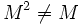$M^2 \ne M$
• The set of minimal prime ideals of$R$ is finite (This needs to be clarified/explained properly)

Now, suppose$M$ were contained in the union of$M^2$ and the minimal prime ideals. Then, by the prime avoidance lemma,$M$ must be contained either in$M^2$ or in one of the minimal prime ideals.$M^2 \ne M$ thus forces$M$ to be a minimal prime ideal, which would make the Krull dimension zero, contradicting our assumption that the Krull dimension is at least 1.

Thus, there exists an element$x$ in$M$ which is outside the union of$M^2$ and all the minimal prime ideals. Let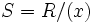$S = R/(x)$ and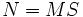$N = MS$. Clearly$N$ is the unique maximal ideal in$S$. By the choice of$S$,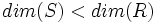$dim(S) < dim(R)$, and in fact we can conclude that$dim(S) = d - 1$ This needs to be clarified/explained properly.

Now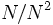$N/N^2$ is a proper homomorphic image of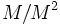$M/M^2$ so it can be generated by$(d-1)$ elements. By Nakayama's lemma,$N$ can also be generated by$(d-1)$ elements.

Thus$S$ is a regular local ring, and hence, by the induction step assumption,$S$ is an integral domain. Hence$x$ is a prime ideal of$R$. But since$x$ lies outside every minimal prime ideal, there is a minimal prime ideal properly contained inside$x$. Call this minimal prime ideal$Q$.

If$y \in Q$ is any element, then we may write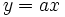$y = ax$ for some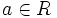$a \in R$. But then since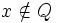$x \notin Q$,$a \in Q$. Thus,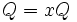$Q = xQ$. Nakayama's lemma now yields$Q = 0$, and hence$R$ is an integral domain.